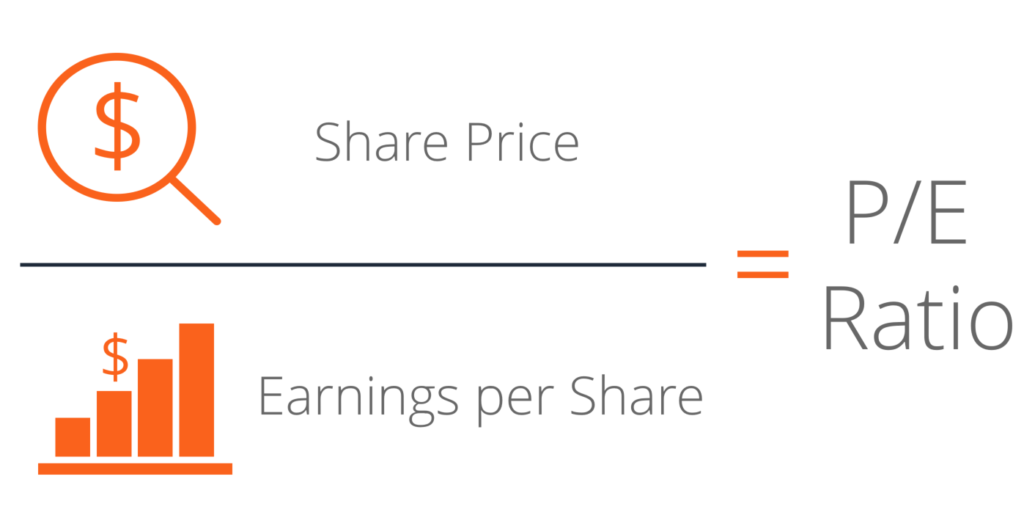# Value Investing : How to Calculate Price to Earnings Ratio of a Company

## Price to Earnings Ratio (P/E ratio)

This is a valuation ratio by this we can know that the share prices are overvalued or undervalued. Formula to calculate P/E ratio:

P/E ratio = Share price / EPS (earning per share).

EPS = Net profit / Total number of outstanding shares

EPS: If a company shares all of its profit to the shareholders then the money that shareholders get per share is called earning per share.

Total outstanding shares = Market cap. / Current share price.

We don’t need to calculate EPS and P/E ratio because we can easily get it on websites like moneycontrol.com.

Now, let’s take an example to know how we can use the P/E ratio to find a company’s valuation.

### Example to find company valuation through P/E ratio

At the point of time while I am writing this course the P/E ratio of HDFC Bank is 29.85. So, is it high or low?

To know that, we have to compare that company’s P/E ratio with other companies of the same industry.

HDFC bank belongs to the bank industry so we compare the P/E ratios of HDFC bank with other bank’s P/E ratios otherwise we can compare it with the P/E ratio of industry (average P/E ratio) which is easily available on websites.

Those companies whose P/E ratio is more than the P/E ratio of it’s industry then the company is overvalued. And if the company’s P/E ratio is less than its industries’ P/E ratio then it’s not only indicated that the company is undervalued it may have some other reasons also like the company is not doing well. And also if the company is at the stage of bankruptcy then it also has a low P/E ratio.

P/E ratio only gives you an overview of the valuation of the company.

If the company is undervalued according to the P/E ratio then there can be many reasons like the company is not showing the actual profits, not doing well, near to bankruptcy, etc. After valuation by the P/E ratio, you have to analyze the reason behind the valuation.

The valuation of the P/E ratio is according to the current performance and not by future planning. And your investments depend on the future performance of the company.

For doing a proper valuation of any company you have to calculate the intrinsic value of the company.

Intrinsic value is calculated by the DFC method which we’ll discuss ahead in this course.

Previous Chapter